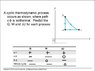# PV Diagram

Punkyc7
Can some one check my work, I'm not sure if I am understanding how internal energy heat and work are related.

Work is defined to be positive if the system does work on the environment.

Q=$\Delta U$ +W

I am showing if the process is positive, negative or 0.

Also this is suppose to be an ideal gas

#### Attachments

•CyclicProcces.jpg
35.2 KB · Views: 403
Last edited:

Homework Helper
Can some one check my work, I'm not sure if I am understanding how internal energy heat and work are related.

Work is defined to be positive if the system does work on the environment.

Q=$\Delta U$ +W

I am showing if the process is positive, negative or 0.

Also this is suppose to be an ideal gas